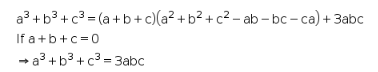# A+b+c=0,then proov a3+b3+c3=3abc

a+b+c=0,then proov a3+b3+c3=3abc